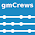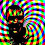## Friday, December 11, 2009

### Dueling Bayesians

Percontations: The Nature of Probability

Interest points:

• Fun with coin-flipping (13:43)

• Can probabilistic thinking be completely automated? (04:31)

• The limits of probability theory (11:07)

• How Andrew got shot down by Daily Kos (06:55)

This part of the discussion was very brief, but probably the most interesting. What Gelman is referring to is maximum entropy sampling, or optimal sequential design of experiments. This has some cool implications for model validation I think (see below).

• The difference between Eliezer and Nassim Taleb (06:20)

Some things Dr Gelman said that I think are interesting:

I was in some ways thinking like a classical statistician, which was, well,I'll be wrong 5% of the time, you know, that's life. We can be wrong a lot, but you're never supposed to knowingly be wrong in Bayesian statistics. If you make a mistake, you shouldn't know that you made a mistake, that's a complete no-no.

With great power comes great responsibility. [...] A Bayesian inference can create predictions of everything, and as a result you can be much more wrong as a Bayesian than as a classical statistician.

When you have 30 cases your analysis is usually more about ruling things out than proving things.

Towards the end of the discussion Yudowski really sounds like he's parroting Jaynes (maybe they are just right in the same way).

### Bayesian design of validation experiments

As I mentioned in the comments about the Gelman/Yudowski discussion, the most interesting thing to me was the ’adaptive testing’ that Gelman mentioned. This is a form of sequential design of experiments , and the Bayesian versions are the most flexible. That is because Bayes theorem provides a consistent and coherent (if not always conveneint) way of updating our knowledge state as each new test result arrives. Then, and Gelman’s comment about ’making predictions about everything’ is germane here, we assess our predictive distributions and find the areas of our parameter space that have the most uncertainty (highest entropy of the predictive distribution). This place in our parameter space with the highest predictive distribution entropy is where we should test next to get the most information. The example of academic testing that Gelman gives does exactly that, the question chosen is the one that the test-taker has equal chance of getting right or wrong.

The same idea applies to testing to validate models. Here’s a little passage from a relevant paper that provides some background and motivation :

Under the constraints of time, money, and other resources, validation experiments often need to be optimally designed for a clearly defined purpose, namely computational model assessment. This is inherently a decision theoretic problem where a utility function needs to be first defined so that the data collected from the experiment provides the greatest opportunity for performing conclusive comparisons in model validation.

The method suggested to achieve this is based on choosing a test point from the area of the parameter space with the highest predictive entropy and also one from the area with the lowest predicitive entropy . This addresses the little comment Gelman made about not being able to asses the goodness of the model very well if you only choose points in the high entropy area. Each round of two test points gives you an opportunity to make the most conclusive comparison of the model prediction to reality.

If you were just trying to calibrate a model, then you would only want to choose test points in the high-entropy-areas because these would do the most to reduce your uncertainty about the reality of interest (and hence give you better parameter estimates). Since we are trying to validate the model though, we want to evaluate its performance where we expect it to give the best predictions and where we expect it to give the worst predictions. Here the idea explained in a bit more technical language :

Consider the likelihood ratio Λ(y) in Eq. (9) [or Bayes factor in ] as a validation metric. Suppose an experiment is conducted with the minimization result, and the experimental output is compared with model prediction. We expect a high value Λ(y)min , where the subscript min indicates that the likelihood is obtained from the experimental output in the minimization case. If Λ(y)min < η, then clearly this experiment rejects the model, since the validation metric Λ(y), even under the most favorable conditions, does not meet the threshold value η. On the other hand, suppose an experiment is conducted with the maximization result, and the experimental output is compared with the model prediction. We expect a low value Λ(y)max < η in this case. If Λ(y)max > η, then clearly this experiment accepts the model, since it is performed under the worst condition and still produces the validation metric to be higher than η. Thus, the cross entropy method provides conclusive comparison as opposed to an experiment at any arbitrary point.

Here's a graphical depiction of the placement of the optimal Bayesian decision boundary (image taken from ):It would be nice to see these sorts of decision theory concepts applied to the public policy decisions that are being driven by the output of computational physics codes.

### References

 Jiang, X., Mahadevan, S., “Bayesian risk-based decision method for model validation under uncertainty,” Reliability Engineering & System Safety, No. 92, pp 707-718, 2007.

 Jiang, X., Mahadevan, S., “Bayesian cross entropy methodology for optimal design of validation experiments,” Measurement Science & Technology, 2006.

 Jiang, X., Mahadevan, S., “Bayesian validation assessment of multivariate computational models ,” Journal of Applied Statistics, Vol. 35, No. 1, Jan 2008.

1.You state that "It would be nice to see these sorts of decision theory concepts applied to the public policy decisions that are being driven by the output of computational physics codes."

I am a bit confused by this. Shouldn't these concepts be applied at the model verification/validation stage? Before the policy decision makers get the models' outputs?

Also, I would be interested in knowing if you have thought about how you would apply these concepts if it were up to you for, say, the climate models.

George

2.gmcrews said: "Shouldn't these concepts be applied at the model verification/validation stage?"

Absolutely.

"Before the policy decision makers get the models' outputs?"

Yes, but also after. Here again Gelman's quote about 'predicting everything' comes into play (it's not either/or, it's both). Once you've decided that a model is 'validated enough' for a particular use, then decision theory gets applied again based on the predictive distributions for the costs (this requires an economic model that takes the predictive distributions from the climate model to predictive distributions for the costs).

I like this approach to policy (deciding what resources to allocate to avoid uncertain costs) because our uncertainty about the underlying physical / economic system is explicitly taken into account. Such a policy response based on decision theory would be coherent (consistent with our state of knowledge of the future 'payouts'), and it would tend to avoid foolish extremes.

"...how you would apply these concepts if it were up to you for, say, the climate models."

Those papers about Bayes Climate Model Averaging are a start. BMA results in a predictive distribution (from an ensemble of climate models), so in principle you could apply the ideas demonstrated in the Xiang & Mahadevan papers with their structural/reliability models to validate the predictions of an ensemble of climate models.

The results of one of those papers cited in the BMA post show that we've still got some systematic biases to iron out. Lindzen & Choi's recent paper is a promising development. Comparing the ensemble performance to the satellite data (of all sorts, launch more sensors!) will bear lots of fruit (proxy and ground station data are too uninformative/low-fidelity to support much further progress).

It is a shame that the modellers and the experimentalists in this field seem to be so adversarial. The aerodynamics community went through those growing pains with the early development of CFD, but thankfully people realized that simulation and experimentation are mutually dependant, and focusing on one at the expense of the other is detrimental to progress in the field as a whole.

3."sometimes the most important thing to come out of an inference is the rejection of the model on which it is based" -- Andrew Gelman